Vinta Software: DjangoCon US 2019: Python & Django in San Diego!

We are back to San Diego!! Our team will be joining DjangoCon US’s conference, one of the biggest Django events in the world. For this year, we’ll be giving two talks: Pull Requests: Merging good practices into your project and Building effective Django queries with expressions Here is the slide from the talk we gave during the conference: Pull Re
Planet Python

Robin Wilson: I am now a freelancer in Remote Sensing, GIS, Data Science & Python

I’ve been doing a bit of freelancing ‘on the side’ for a while – but now I’ve made it official: I am available for freelance work. Please look at my new website or contact me if you’re interested in what I can do for you, or carry on reading for more details.

Since I stopped working as an academic, and took time out to focus on my work and look after my new baby, I’ve been trying to find something which allows me to fit my work nicely around the rest of my life. I’ve done bits of short part-time work contracts, and various bits of freelance work – and I’ve now decided that freelancing is the way forward.

I’ve created a new freelance website which explains what I do and the experience I have – but to summarise here, my areas of focus are:

• Remote Sensing – I am an expert at processing satellite and aerial imagery, and have processed time-series of thousands of images for a range of clients. I can help you produce useful information from raw satellite data, and am particularly experienced at atmospheric remote sensing and atmospheric correction.
• GIS – I can process geographic data from a huge range of sources into a coherent data library, perform analyses and produce outputs in the form of static maps, webmaps and reports.
• Data science – I have experience processing terabytes of data to produce insights which were used directly by the United Nations, and I can apply the same skills to processing your data: whether it is a single questionnaire or a huge automatically-generated dataset. I am particularly experienced at making research reproducible and self-documenting.
• Python – I am an experienced Python programmer, and maintain a number of open-source modules (such as Py6S). I produce well-written, Pythonic code with high-quality tests and documentation.

The testimonials on my website show how much previous clients have valued the work I’ve done for them.

I’ve heard from a various people that they were rather put off by the nature of the auction that I ran for a day’s work from me – so if you were interested in working with me but wanted a standard sort of contract, and more than a day’s work, then please get in touch and we can discuss how we could work together.

(I’m aware that the last few posts on the blog have been focused on the auction for work, and this announcement of freelance work. Don’t worry – I’ve got some more posts lined up which are more along my usual lines. Stay tuned for posts on Leaflet webmaps and machine learning of large raster stacks)

Planet Python

Erik Marsja: How to Read & Write SPSS Files in Python using Pandas

The post How to Read & Write SPSS Files in Python using Pandas appeared first on Erik Marsja.

In this post we are going to learn 1) how to read SPSS (.sav) files in Python, and 2) how to write to SPSS (.sav) files using Python.

Python is a great general-purpose language as well as for carrying out statistical analysis and data visualization. However, Python is not really user-friendly for data storage. Thus, often our data will be archived using Excel, SPSS or similar software.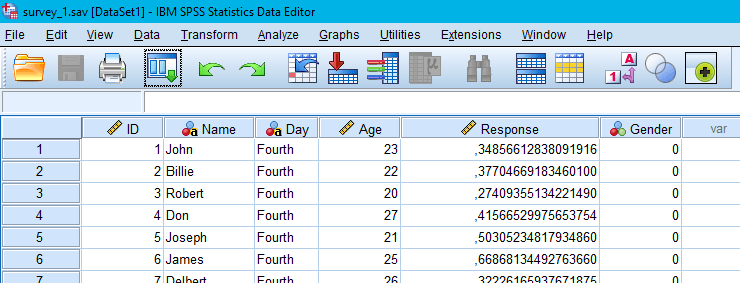How to open a .sav file in Python? There are some packages as Pyreadstat, and Pandas which allow to perform this operation. If we are working with Pandas, the   read_spss method will load a .sav file into a Pandas dataframe. Note, Pyreadstat will also create a Pandas dataframe from a SPSS file.

How to Open a SPSS file in Python

Here’s two simple steps on how to read .sav files in Python using Pandas (more details will be provided in this post):

1. import pandas

in your script type “import pandas as pd

in your script use the read_spss method:

In this secion, we are going to learn how to load a SPSS file in Python using the Python package Pyreadstat. Before we use Pyreadstat we are going to install it. This Python package can be installed in two ways.

How to install Pyreadstat:

There are two very easy methods to install Pyreadstat.:

1. Install Pyreadstat using pip:
Open up a terminal, or windows command prompt, and type pip install pyreadstat
2. Install using Conda:
Open up a terminal, or windows command prompt, and type conda install -c conda-forge pyreadstat

How to Load a .sav File in Python Using Pyreadstat

Every time we run our Jupyter notebook, we need to load the packages we need. In the, Python read SPSS example below we will use Pyreadstat and, thus, the first line of code will import the package:

Now, we can use the method read_sav to read a SPSS file. Note that, when we load a file using the Pyreadstat package, recognize that it will look for the file in Python’s working directory. In the read SPSS file in Python example below, we are going to use this SPSS file. Make sure to download it and put it in the correct folder (or change the path in the code chunk below):

In the code chunk above we create two variables; df, and meta. As can be seen when using type df is a Pandas dataframe:

type(df)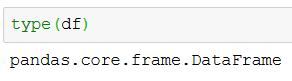Thus, we can use all methods available for Pandas dataframe objects. In the next line of code, we are going to print the 5 first rows of the dataframe using pandas head method.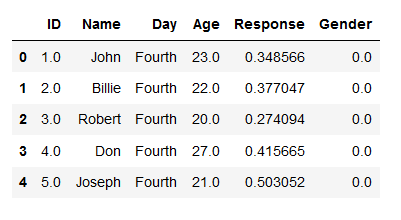See more about working with Pandas dataframes in the following tutorials:

How to Read a SPSS file in Python Using Pandas

Pandas can, of course, also be used to load a SPSS file into a dataframe. Note, however, we need to install the Pyreadstat package as, at least right now, Pandas depends on this for reading .sav files. As always, we need to import Pandas as pd:

import pandas as pd

Now, when we have done that, we can read the .sav file into a Pandas dataframe using the read_spss method. In the read SPSS example below, we read the same data file as earlier and print the 5 last rows of the dataframe using Pandas tail method. Remember, using this method also requires you to have the file in the subfolder “simData” (or change the path in the script).

df = pd.read_spss('./SimData/survey_1.sav') df.tail()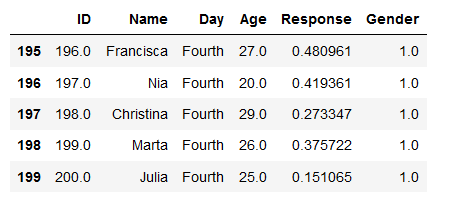Note, that both read_sav (Pyreadstat) and read_spss have the arguments “usecols”. By using this argument, we can also select which columns we want to load from the SPSS file to the dataframe:

cols = ['ID', 'Day', 'Age', 'Response', 'Gender'] df = pd.read_spss('./SimData/survey_1.sav', usecols=cols) df.head()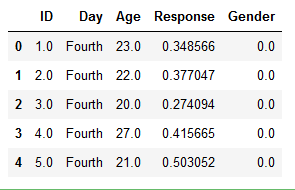How to Write a SPSS file Using Python

Now we are going to learn how to save Pandas dataframe to a SPSS file. It’s simpe, we will use the Pyreadstats write_sav method. The first argument should be the Pandas dataframe that is going to be saved as a .sav file.

Remember to put the right path, as second argument, when using write_sav to save a .sav file.
Unfortunately, Pandas don’t have a to_spss method, yet. But, as Pyreadstats is a dependency of Pandas read_spss method we can use it to write a SPSS file in Python.

Summary: Read and Write .sav Files in Python

Now we have learned how to read and write .sav files using Python. It was quite simple and both methods are, in fact, using the same Python packages.

Here’s a Jupyter notebook with the code used in this Python SPSS tutorial.

The post How to Read & Write SPSS Files in Python using Pandas appeared first on Erik Marsja.

Planet Python

Real Python: Python Histogram Plotting: NumPy, Matplotlib, Pandas & Seaborn

In this course, you’ll be equipped to make production-quality, presentation-ready Python histogram plots with a range of choices and features.

If you have introductory to intermediate knowledge in Python and statistics, then you can use this article as a one-stop shop for building and plotting histograms in Python using libraries from its scientific stack, including NumPy, Matplotlib, Pandas, and Seaborn.

A histogram is a great tool for quickly assessing a probability distribution that is intuitively understood by almost any audience. Python offers a handful of different options for building and plotting histograms. Most people know a histogram by its graphical representation, which is similar to a bar graph: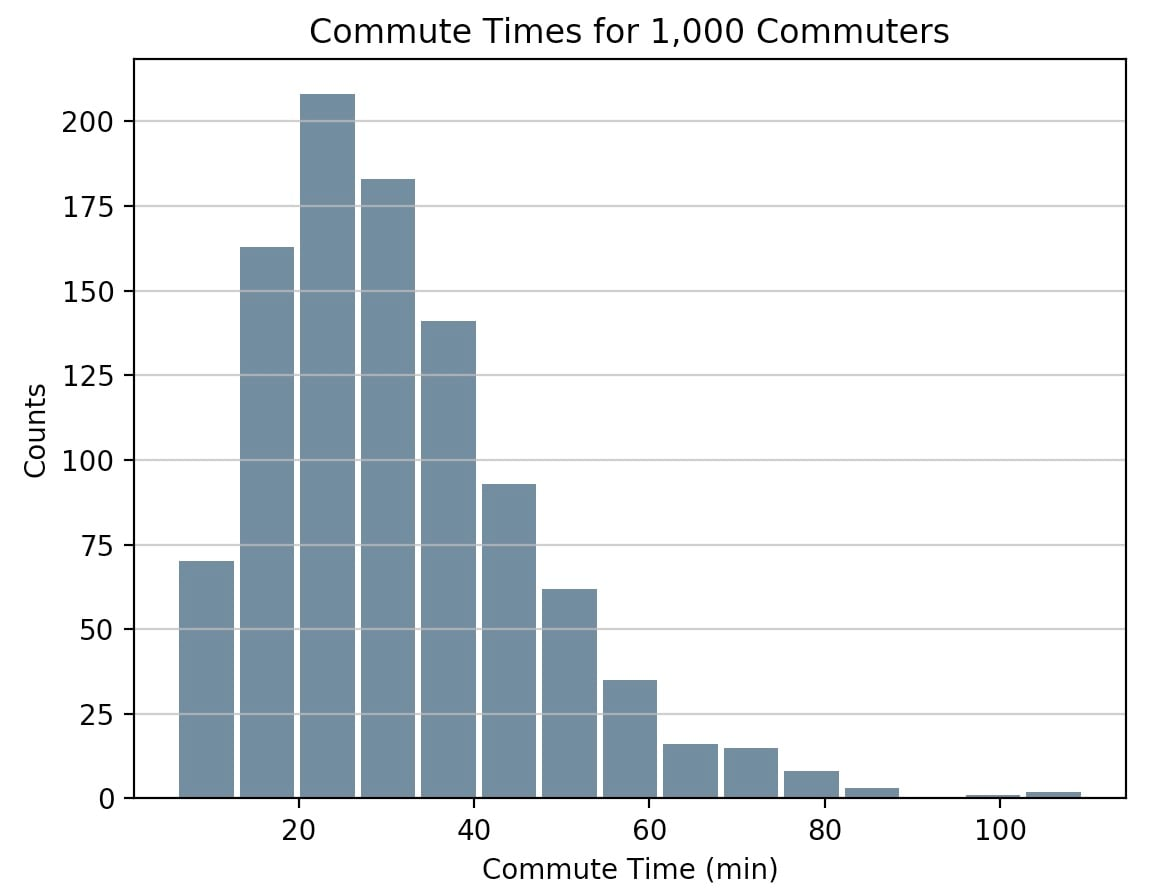This course will guide you through creating plots like the one above as well as more complex ones. Here’s what you’ll cover:

• Building histograms in pure Python, without use of third party libraries
• Constructing histograms with NumPy to summarize the underlying data
• Plotting the resulting histogram with Matplotlib, Pandas, and Seaborn

Free Bonus: Short on time? Click here to get access to a free two-page Python histograms cheat sheet that summarizes the techniques explained in this tutorial.

[ Improve Your Python With 🐍 Python Tricks 💌 – Get a short & sweet Python Trick delivered to your inbox every couple of days. >> Click here to learn more and see examples ]

Planet Python

Erik Marsja: Repeated Measures ANOVA in R and Python using afex & pingouin

In this post we will learn how to carry out repeated measures Analysis of Variance (ANOVA) in R and Python. To be specific, we will use the R package afex and the Python package pingouin to carry out one-way and two-way ANOVA f or within subject’s design. The structure of the following data analysis tutorial is as follows; a brief introduction to (repeated measures) ANOVA, carrying out within-subjects ANOVA in R using afex and in Python using pingouin. In the end, there will be a comparison of the results and the pros and cons using R or Python for data analysis (i.e., ANOVA).

What is ANOVA?

Before we go into how to carry out repeated measures ANOVA in R and Python, we are briefly going to learn what an ANOVA is. An ANOVA test is a parametrical method to find out whether the results from collected data are significant. That is, this type of test will enable us to figure out whether we should to reject the null hypothesis or accept the alternate hypothesis. In a between ANOVA we’re testing groups to see if there’s a statistical difference between them. In this post we are going to learn to do repeated measures ANOVA, however, and using this method we compare means across one or more variables that are based on repeated observations. These repeated observations can either be time points or different conditions. In the repeated ANOVA examples below we use different conditions.

Data

In this repeated measures ANOVA example, we will use fake data (can be downloaded here). This fake data is a sample of 60 adults responding as fast as they can to visual stimuli. This, the dependent variable (DV) is response time to the visual stimuli. While the subjects were categorizing visual stimuli, they were either exposed to background noise or quiet (independent variable, iv1).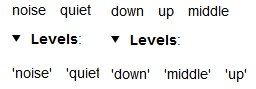In the first example, we are going to use these two conditions (iv1) when we carry out a one-way ANOVA for repeated measures. Furthermore, the visual stimuli could either be presented in the upper part, lower part, or in the middle part of the computer screen (independent variable, iv2).

The variables given in the data set:

• Sub_id = Subject ID #
• iv1 = Noise condition; quiet or noise
• iv2 = Location condition; upper, lower, middle
• DV = response time

Repeated Measures ANOVA in R

In this section we are going to learn how to do a repeated measures ANOVA in R using afex. More specifically, we are going to learn how carry out a one-way and two-way ANOVA using the aov_ez function. Note, working with aov_ez function we need to have our data in long format.

Installing afex

First, we are going to install the needed package: afex. In the code chunk, below , the package will only be installed if it’s not already installed.

list.of.packages <- c("afex", "emmeans") new.packages <- list.of.packages[!(list.of.packages %in% installed.packages()[,"Package"])] if(length(new.packages)) install.packages(new.packages)

One-Way Repeated Measures ANOVA in R.

In the first example, we are going to carry out a one-way repeated measures ANOVA in R using aov_ez. Here we want to know whether there is any difference in response time with background noise compared to without background noise. To test this, we need to conduct a within-subjects ANOVA.

In the first code chunk, below, we load the package, the data, and print the first 5 rows using head

Example ANOVA for Within-Subjects Design:

aov <- aov_ez('Sub_id', 'rt',               fun_aggregate = mean, df, within = 'iv1') print(aov)

Two-Way Repeated Measures ANOVA in R

In the second example, we are going to conduct a two-way repeated measures ANOVA in R. Here we want to know whether there is any difference in response time during background noise compared to without background noise, and whether there is a difference depending on where the visual stimuli are presented (up, down, middle). Finally, we are interested if there is an interaction between the noise and location conditions.

aov <- aov_ez('Sub_id', 'rt', fun_aggregate = mean,               df, within = c('iv1', 'iv2')) print(aov)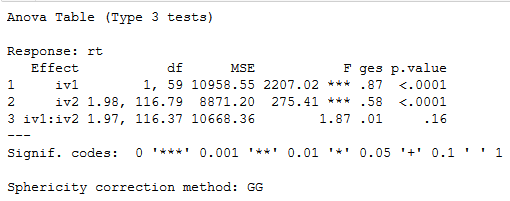Plotting an Interaction

The R package afex also have a function to plot an interaction. Now, before continuing with the Python ANOVA, we are going to use this function.

afex_plot(aov, x = "iv1", trace = "iv2",          error = "within")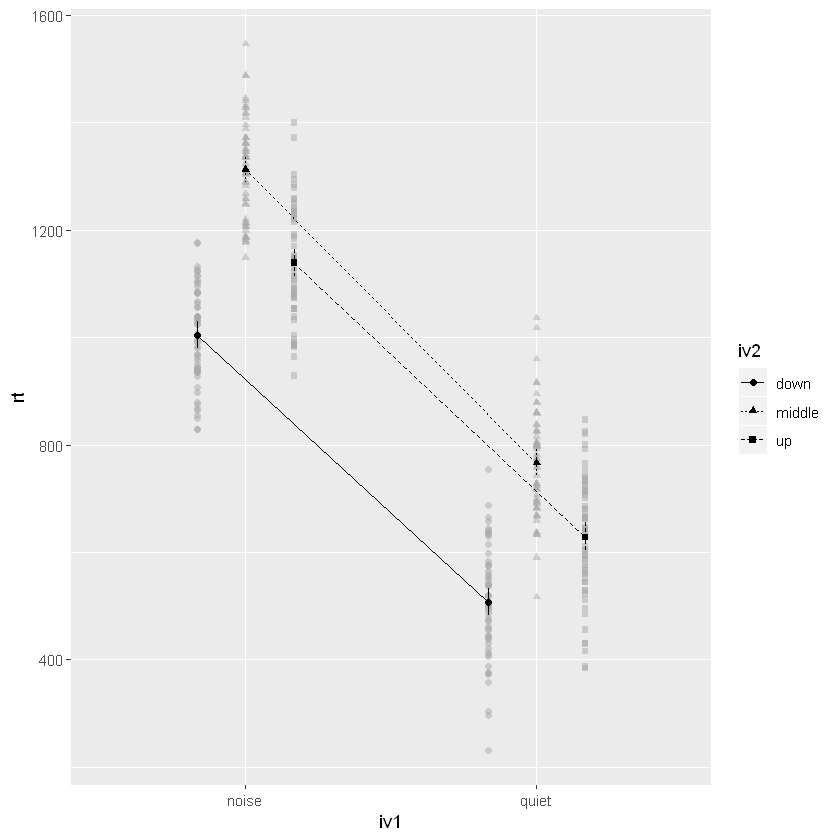As can be seen, and confirmed by the ANOVA table above, we see that there is no interaction. If we had an interaction, we could follow this up with pairwise comparisons using the package emmeans.

Here’s a Jupyter Notebook containing the above code examples.

Repeated Measures ANOVA in Python

Now that we know how to conduct a within-subjects ANOVA in R we are going to carry out the same ANOVA in Python. In a previous post, we learned how to use the class AnovaRM from the Python package Statsmodels. In this post, however, we are going to use the package pingouin and the function anova_rm. Note, this function can handle both a wide and a long format data file.

One-Way Repeated Measures ANOVA in Python

In the first example, we are going to conduct a one-way ANOVA for repeated measures using Python. We start by imporring pandas as pd and pingoin as pg:

import pandas as pd import pingouin as pg   df = pd.read_csv('./Python_ANOVA/rmAOV2way.csv') df.head()

Learn more about how to work with Pandas dataframe and load data from different file types:

Now we can carry out our repeated measures ANOVA using Python:

aov = pg.rm_anova(dv='rt', within='iv1',                    subject='Sub_id', data=df, detailed=True) print(aov.round(2))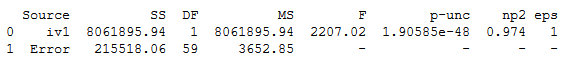Two-Way Repeated Measures ANOVA in Python

In the second example, we are going to carry out a two-way ANOVA for repeated measures using Python.

aov = pg.rm_anova(dv='rt',                    within=['iv1', 'iv2'],                    subject='Sub_id', data=df) print(aov.round(2))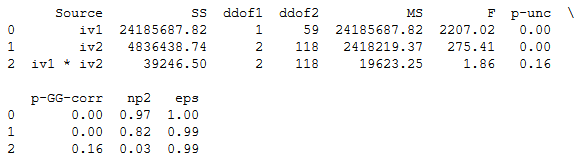Interaction Plot in Python using Seaborn

For completeness, even though we didn’t have a significant interaction, we are going to create an interaction plot using Seaborn:

import seaborn as sns  ax = sns.pointplot(x="iv1", y="rt", hue="iv2",                     data=df)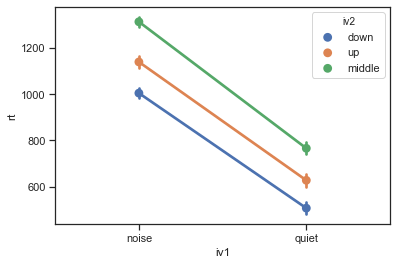Pingouin also comes with a function to carry out pairwise comparison. If we had a significant interaction, we could use it. See this post for an example how to use this function.

Here’s a Jupyter Notebook containing the Python ANOVA examples above.

Conclusion: R vs Python

In this post, we have learned how to carry out one-way and two-way ANOVA for repeated measures using R and Python. We have used the r-package afex and the Python package pingouin. Both afex and pingouin are quite similar; they offer the Greenhouse-Geisser correction. In afex, however, you can c hoose to get either partial eta-squared or general eta-squared effect sizes. Furthermore, as can be seen in the ANOVA tables the results are basically the same.

In conclusion, the packages afex and pingouin offers an easy way to carry out ANOVA for within-subject designs in R and Python, respectively.

Resources

Here are some previous posts on how to carry out ANOVA in Python:

The post Repeated Measures ANOVA in R and Python using afex & pingouin appeared first on Erik Marsja.

Planet Python